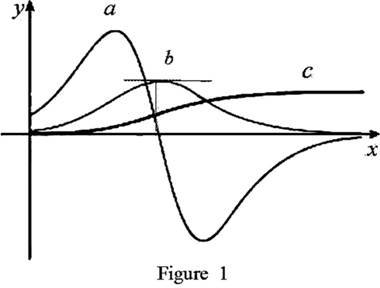# Each curves on the given graph and give proper explanation.### Single Variable Calculus: Concepts...

4th Edition
James Stewart
Publisher: Cengage Learning
ISBN: 9781337687805### Single Variable Calculus: Concepts...

4th Edition
James Stewart
Publisher: Cengage Learning
ISBN: 9781337687805

#### Solutions

Chapter 2.7, Problem 43E
To determine

## To identify: Each curves on the given graph and give proper explanation.

Expert Solution

The curve c is position curve, b is velocity curve and a is acceleration curve.

### Explanation of Solution

Graph:

The given graph is shown as in Figure 1,Observation:

Observe the graph of b and a carefully.

The point where a(t)=0 is the same point where graph of b(t) has horizontal tangent.

Recall that the derivative of a function is zero where the function has a horizontal tangent.

Therefore, a(t) is the derivative of the graph b(t). That is, b(t)=a(t).

Observe the graph of a and c carefully.

The graph of a has both positive and negative values. Hence a can be either velocity or acceleration.

The points where the graph of a has horizontal tangent, the functional value of c is not zero at that point.

This implies that, a(t)c(t).

The only possibility is that a is the acceleration curve. This implies that c(t)=a(t).

So, c=b=a.

Thus, c is position curve, b is velocity curve and a is acceleration curve.

### Have a homework question?

Subscribe to bartleby learn! Ask subject matter experts 30 homework questions each month. Plus, you’ll have access to millions of step-by-step textbook answers!